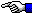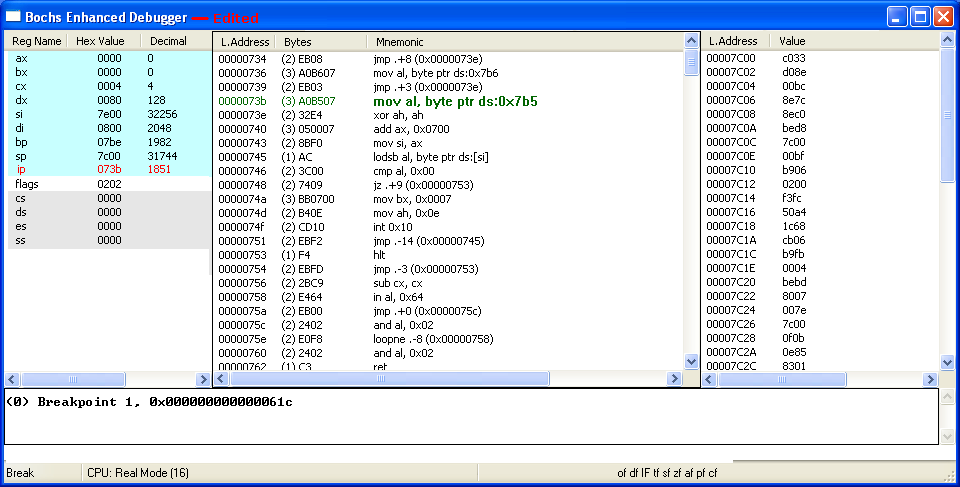Invalid Partition Entry Found
(Pathways through the Windows 7 MBR)

If you need any help in setting up the Bochs Debugger, please email us.Back to: Pathways through the Windows 7 MBR page.

If any of the four 16-byte Partition Table entries (there can only be 4 entries under the standard 'Basic Disk'/MBR Partitioning Scheme) has a 01h through 7Fh byte as its first byte, the MBR code will notify the user it has encountered an "Invalid" partition entry; this will halt execution, even if a valid Active partition entry follows the invalid one! Starting back at instruction 0x623, we show a 01 byte at Memory location 0x7BE:

 ``` The code places 07be into the Base Pointer (BP) Register to check for 80h at the correct offset in the first Partition Table entry: AX=0000 BX=0000 CX=0004 DX=0080 SP=7C00 BP=07BE SI=7E00 DI=0800 DS=0000 ES=0000 SS=0000 CS=0000 IP=0623 of df IF sf ZF af PF cf NV UP EI PL ZR NA PE NC 0623 807E0000 CMP BYTE PTR [BP+00],00 SS:07BE=01```

So our CMP (CoMPare) instruction proceeds by subtracting the second operand (the Zero) from the first operand (the value found at Memory location 0x7BE; the 01h). Since 1 - 0 = 1, there's no change in the Sign Flag; it's still positive (sf=0), but the Zero Flag is reset (zf=0) since the result is not all zero bits and the Parity Flag is also reset (pf=0) since there's a single 1's bit (an odd number) in the result; all other flags are unaffected. This would also be true for any of the other values from 02h through 7Fh; with the only exceptions being those values where the Parity would be even (PF=1): 03, 05, 06, 09, 0Ah, 0Ch, 0Fh, etc. However, parity never affects the pathways taken by this MBR code.

 ```AX=0000 BX=0000 CX=0004 DX=0080 SP=7C00 BP=07BE SI=7E00 DI=0800 DS=0000 ES=0000 SS=0000 CS=0000 IP=0627 of df IF sf zf af pf cf 0627 7C0B JL 0634 NV UP EI PL NZ NA PO NC ```

And since we still have a positive value (sf=0), excution simply proceeds to the next instruction:

 ```AX=0000 BX=0000 CX=0004 DX=0080 SP=7C00 BP=07BE SI=7E00 DI=0800 DS=0000 ES=0000 SS=0000 CS=0000 IP=0629 of df IF sf zf af pf cf 0629 0F850E01 JNZ 073B NV UP EI PL NZ NA PO NC```

Since any value from 01h through 7Fh is both positive (sf=0) and non-zero (zf=0), having such a value as a Partition Table entry's Boot Indicator byte means execution will eventually display the "Invalid partition table" message. So, the JNZ (Jump if Not Zero) instruction jumps to 0x73B:As was shown on our Windows 7 MBR page, the following instructions set up the CPU registers used in displaying the "Invalid partition table" Error Message stored (by this time) at linear Memory location 0x763h (which is also 0000:0763 in terms of Segment:Offset) and following.

 ```AX=0000 BX=0000 CX=0004 DX=0080 SP=7C00 BP=07BE SI=7E00 DI=0800 DS=0000 ES=0000 SS=0000 CS=0000 IP=073B of df IF sf zf af pf cf NV UP EI PL NZ NA PO NC 073B A0B507 MOV AL,[07B5] DS:07B5=63 mov al,[07B5] copies the contents of this Memory location (a 63h in this case) into the Lower half (the L in AL) of the AX Register: AX=0063 BX=0000 CX=0004 DX=0080 SP=7C00 BP=07BE SI=7E00 DI=0800 DS=0000 ES=0000 SS=0000 CS=0000 IP=073E of df IF sf zf af pf cf 073E 32E4 XOR AH,AH NV UP EI PL NZ NA PE NC xor ah, ah doesn't make any real change, since the High byte of AX is already zeroed out: AX=0063 BX=0000 CX=0004 DX=0080 SP=7C00 BP=07BE SI=7E00 DI=0800 DS=0000 ES=0000 SS=0000 CS=0000 IP=0740 of df IF sf zf af pf cf 0740 050007 ADD AX,0700 NV UP EI PL NZ NA PE NC add ax, 0700 results in the Offset 0763h; where MBR Error Messages begin in Memory; this one points to: "Invalid partition table". AX=0763 BX=0000 CX=0004 DX=0080 SP=7C00 BP=07BE SI=7E00 DI=0800 DS=0000 ES=0000 SS=0000 CS=0000 IP=0743 of df IF sf zf af pf cf 0743 8BF0 MOV SI,AX NV UP EI PL NZ NA PE NC mov si, ax sets up the Source Index location for the routine which will eventaully DISPLAY the Error Message on the PC screen: AX=0763 BX=0000 CX=0004 DX=0080 SP=7C00 BP=07BE SI=0763 DI=0800 DS=0000 ES=0000 SS=0000 CS=0000 IP=0745 of df IF sf zf af pf cf 0745 AC LODSB NV UP EI PL NZ NA PE NC```

Location of English Error Messages and
Message Offsets in Memory

 ``` 3 4 5 6 7 8 9 A B C D E F 0763 49 6E 76 61 6C 69 64 20 70 61 72 74 69 Invalid parti 0770 74 69 6F 6E 20 74 61 62 6C 65 00 45 72 72 6F 72 tion Table.Error 0780 20 6C 6F 61 64 69 6E 67 20 6F 70 65 72 61 74 69 loading operati 0790 6E 67 20 73 79 73 74 65 6D 00 4D 69 73 73 69 6E ng system.Missin 07A0 67 20 6F 70 65 72 61 74 69 6E 67 20 73 79 73 74 g operating syst 07B0 65 6D 00 00 00 63 7B 9A em...c{. 0 1 2 3 4 5 6 7 8 9 A B C D E F```

Error Messages Display Routine

This LODSB (LOaD String Byte) instruction causes data to be loaded into the AL register, one byte at a time, from a string of bytes pointed at by the Memory location currently stored in the DS:SI (Data Segment:Source Index) registers. After a byte has been copied into AL, the value in SI will be incremented by 1, since the Direction Flag bit is "UP" (df=0). The routine uses a 'Compare' and 'Conditional Jump' (JZ) to run through a string of bytes; depending on locating a ZERO byte at the end of the string, in order to exit the loop formed by the non-conditional Jump at Memory location 0x751 back to LODSB at 0x745.

Since the AL register will contain whatever byte has just been copied to it by the LODSB instruction, we've decided to represent these varying values with a 'cc' symbol in the figures below; we are not going to step through every character of each possible message string! Likewise, the increasing values of the SI register will be represented by an 'nn' symbol.

 ```AX=07cc BX=0000 CX=0004 DX=0080 SP=7C00 BP=07BE SI=07nn DI=0800 DS=0000 ES=0000 SS=0000 CS=0000 IP=0746 of df IF sf zf af pf cf 0746 3C00 CMP AL,00 NV UP EI PL NZ NA PO NC```

More will be posted in the near future... this page is still Under Construction !!!

If you need any help in setting up the Bochs Debugger, please email us.

First Published: July 5, 2013 (05.07.2013).
Updated:.
Last Update: July 11, 2013. (11.07.2013)

You can write to us using this: online reply form. (It opens in a new window.)Pathways through the Windows 7 MBRThe Starman's x86 ASSEMBLY PagesThe Starman's Realm Index Page